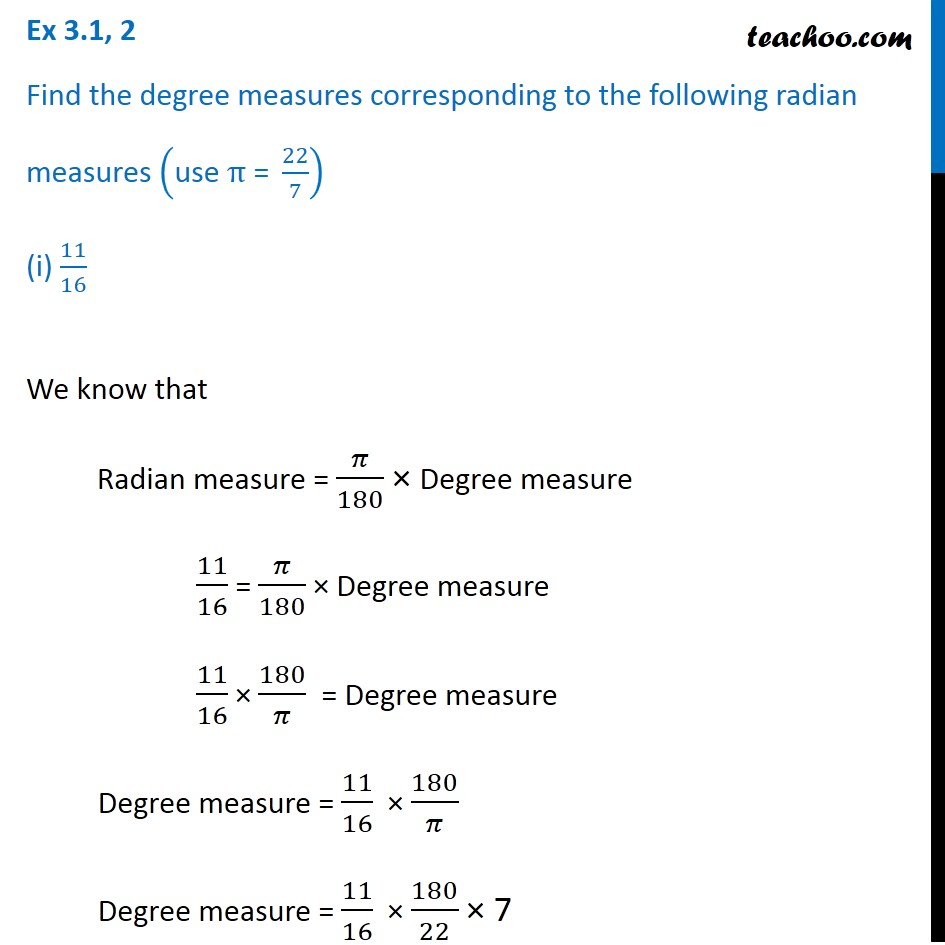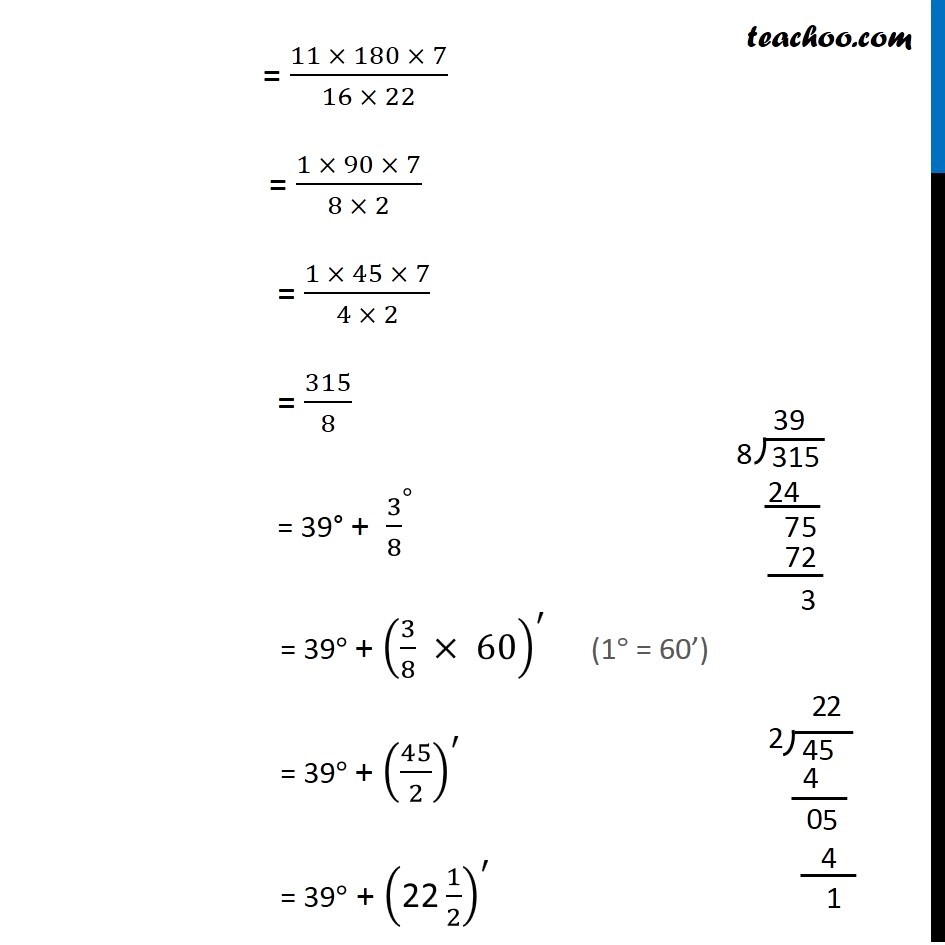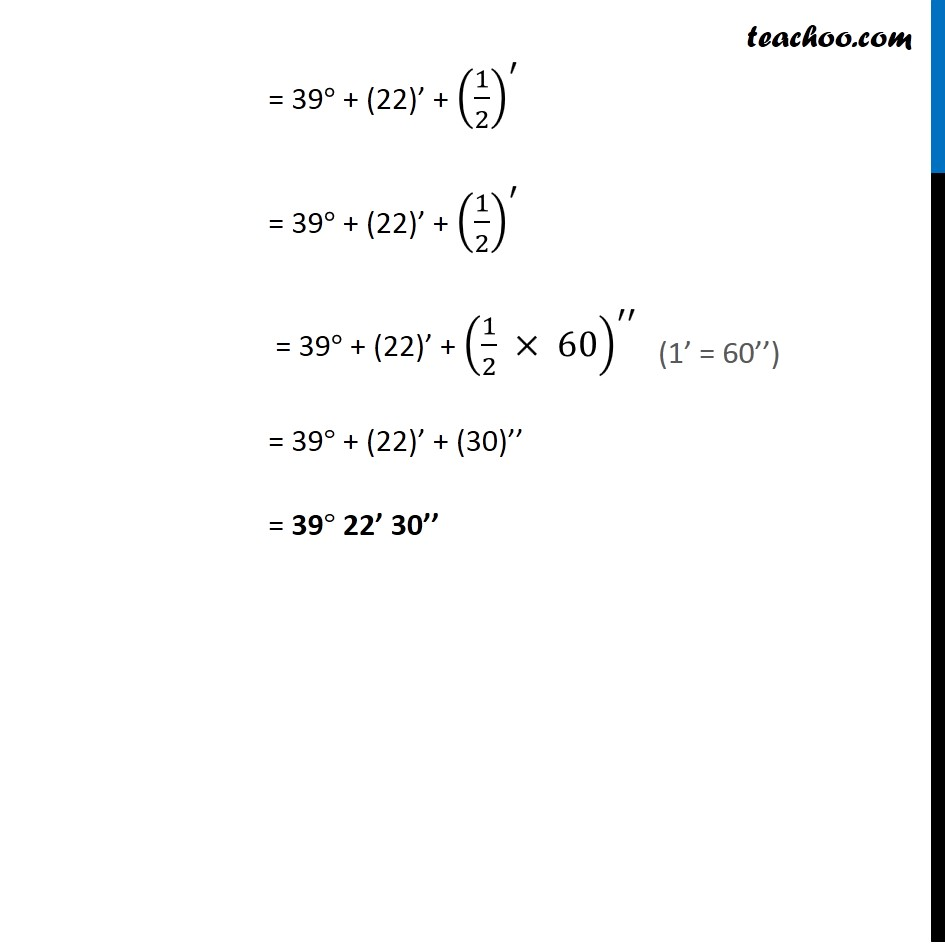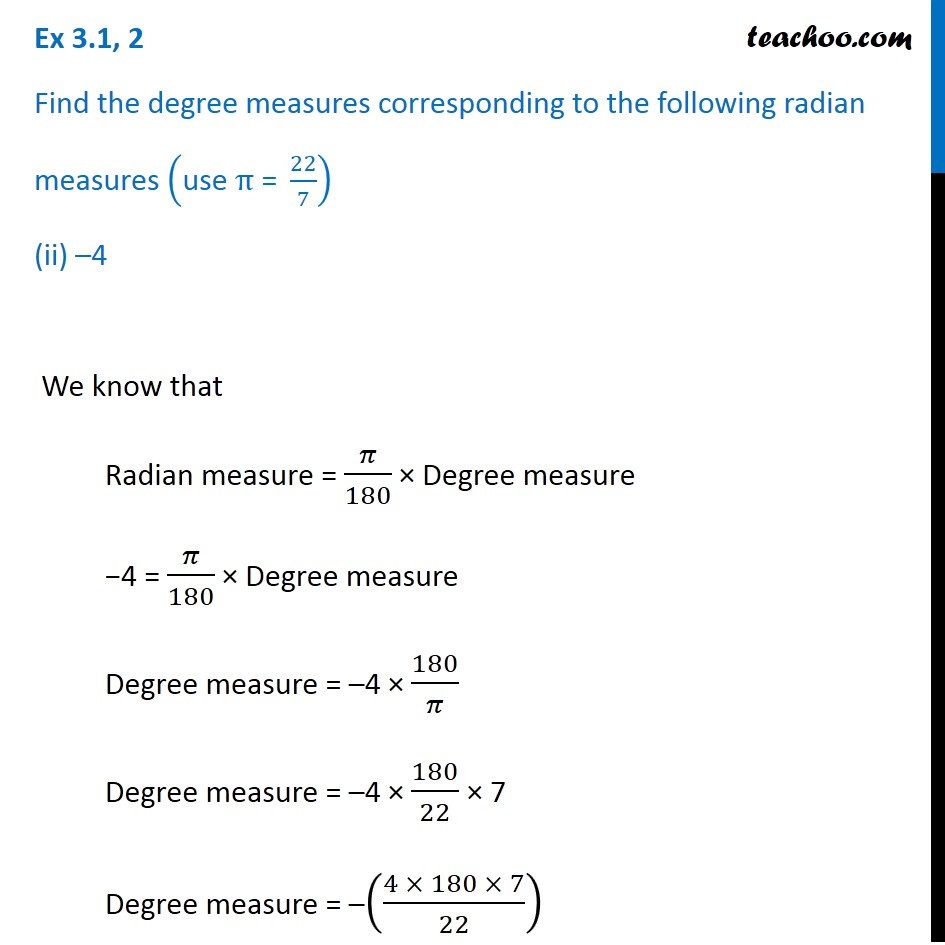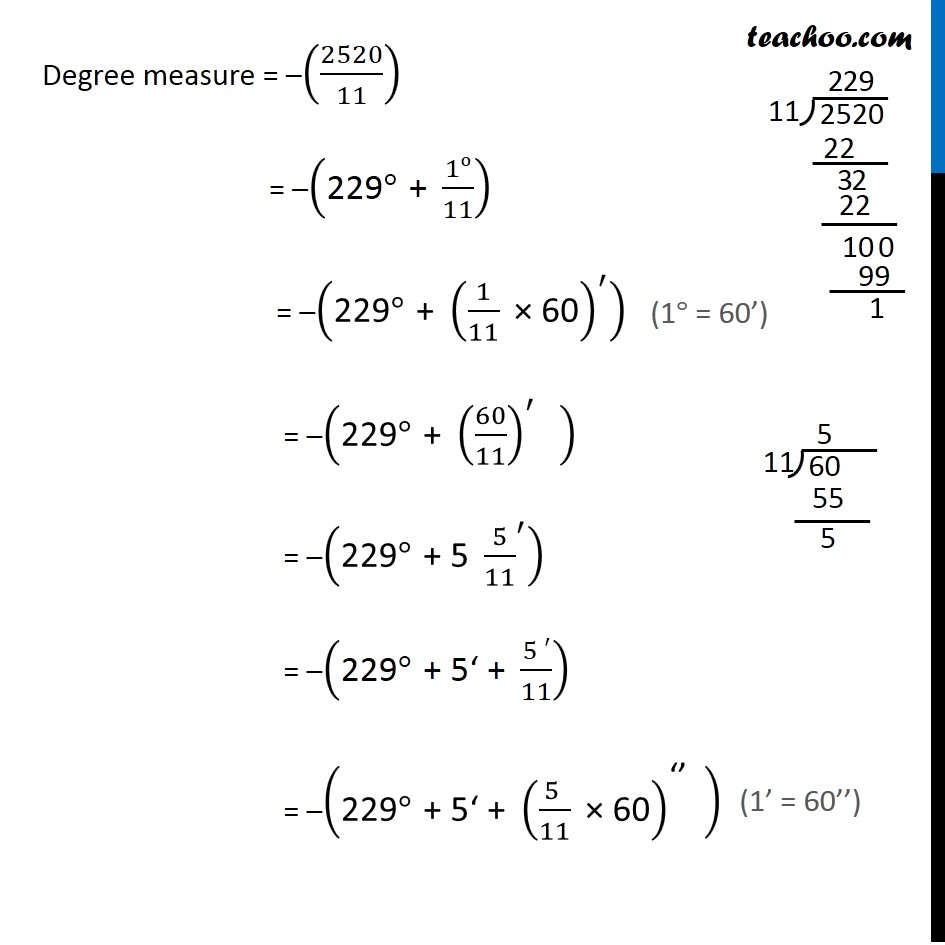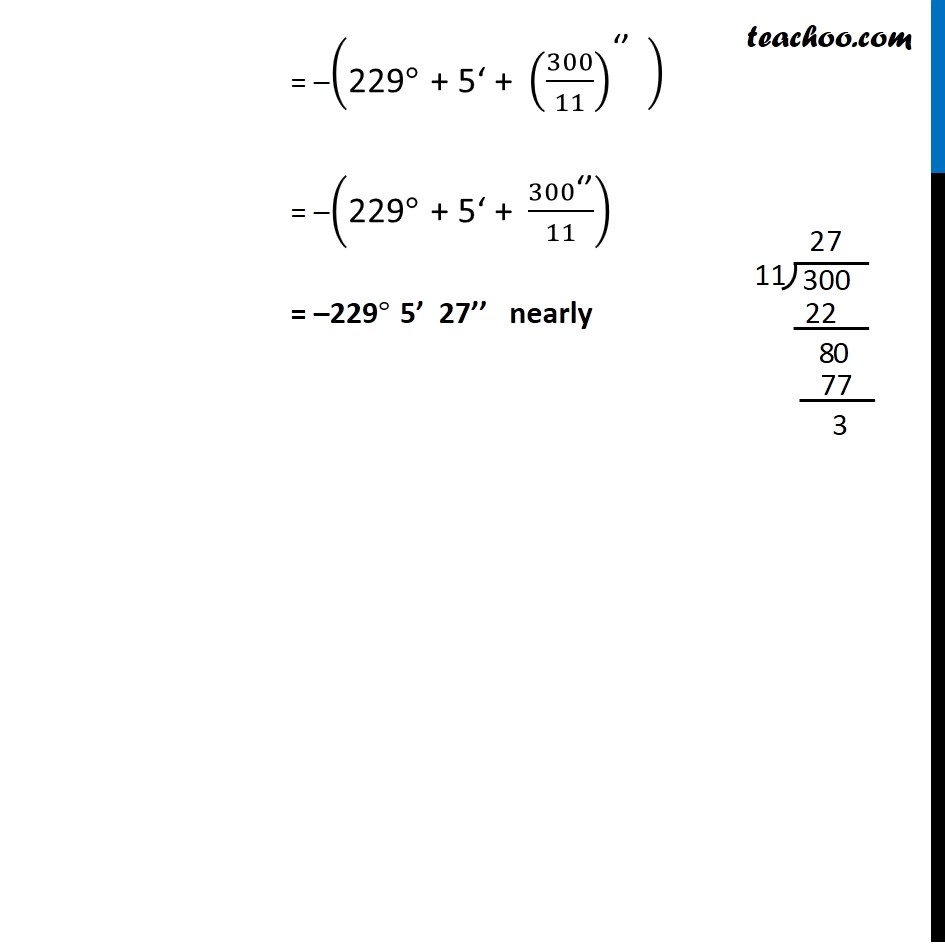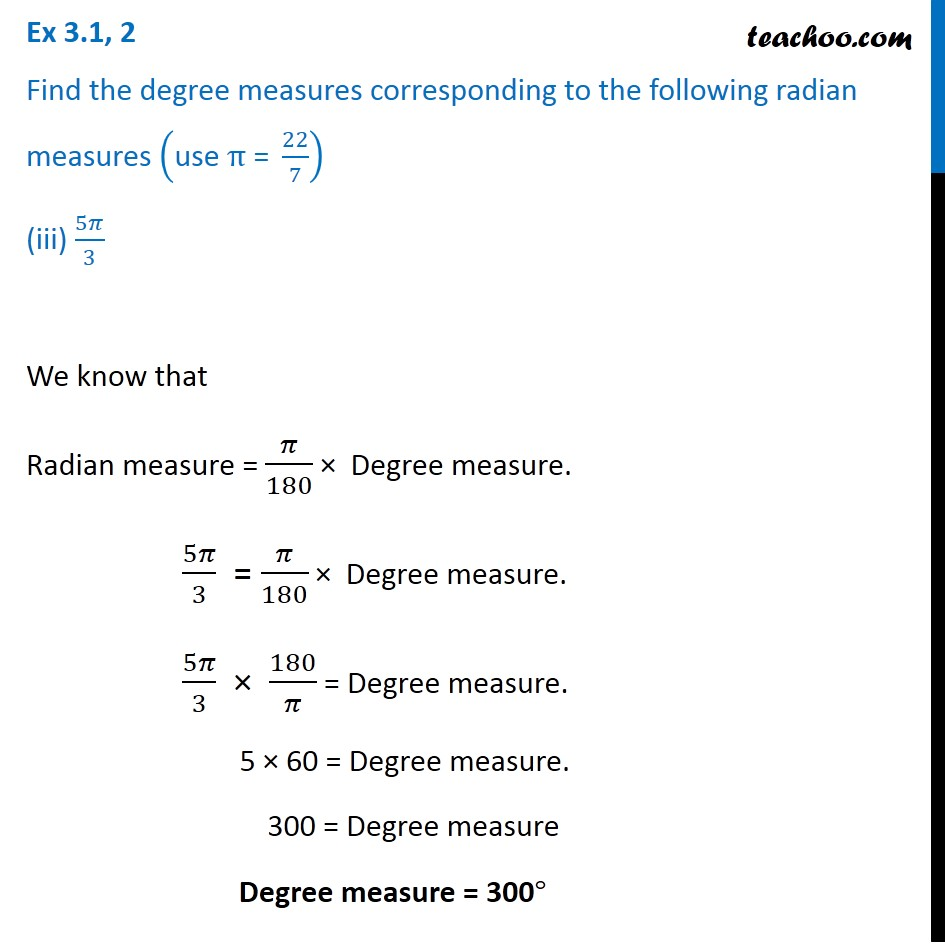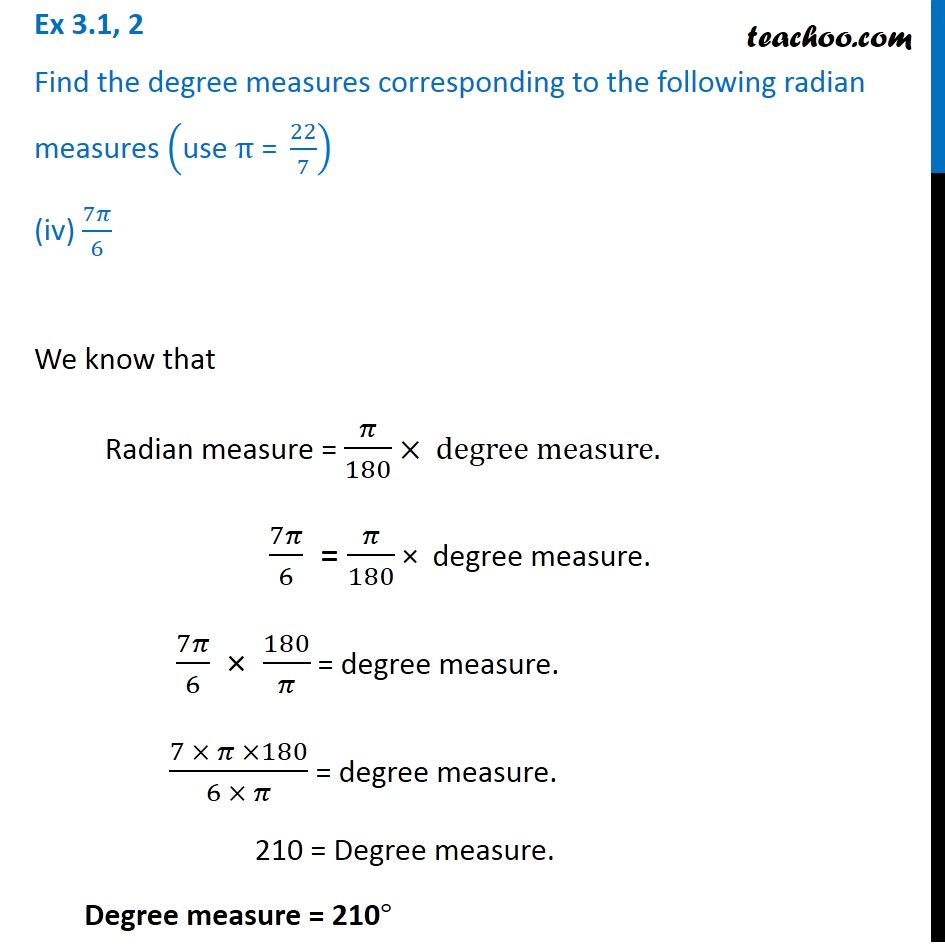1. Chapter 3 Class 11 Trigonometric Functions
2. Serial order wise
3. Ex 3.1

Transcript

Ex 3.1, 2 Find the degree measures corresponding to the following radian measures ("use " π" = " 22/7) (i) 11/16 We know that Radian measure = 𝜋/180 × Degree measure 11/16 = 𝜋/180 × Degree measure 11/16 × 180/𝜋 = Degree measure Degree measure = 11/16 × 180/𝜋 Degree measure = 11/16 × 180/22 × 7 = (11 × 180 × 7)/(16 × 22) = (1 × 90 × 7)/(8 × 2) = (1 × 45 × 7)/(4 × 2) = 315/8 = 39° + 〖3/8〗^° = 39° + (3/8 × 60)^′ = 39° + (45/2)^′ = 39° + ("22" 1/2)^′ = 39° + (22)’ + (1/2)^′ = 39° + (22)’ + (1/2)^′ = 39° + (22)’ + (1/2 × 60)^′′ = 39° + (22)’ + (30)’’ = 39° 22’ 30’’ Ex 3.1, 2 Find the degree measures corresponding to the following radian measures ("use " π" = " 22/7) (ii) –4 We know that Radian measure = 𝜋/180 × Degree measure −4 = 𝜋/180 × Degree measure Degree measure = –4 × 180/𝜋 Degree measure = –4 × 180/22 × 7 Degree measure = –((4 × 180 × 7)/22) Degree measure = –(2520/11) = –("229" °" + " 1o/11) = –("229" °" + " (1/11 " × 60" )^′ ) = –("229" °" + " (60/11)^′ " " ) = –("229" °" + 5 " 〖5/11〗^′ ) = –("229" °" + 5‘ + " (5 ′)/11) = –("229" °" + 5‘ + " ((5 )/11 " × 60" )^"‘’" " " ) = –("229" °" + 5‘ + " (300/11)^"‘’" " " ) = –("229" °" + 5‘ + " 300"‘’" /11) = –229° 5’ 27’’ nearly Ex 3.1, 2 Find the degree measures corresponding to the following radian measures ("use " π" = " 22/7) (iii) 5𝜋/3 We know that Radian measure = 𝜋/180 × Degree measure. 5𝜋/3 = 𝜋/180 × Degree measure. 5𝜋/3 × 180/𝜋 = Degree measure. 5 × 60 = Degree measure. 300 = Degree measure Degree measure = 300° Ex 3.1, 2 Find the degree measures corresponding to the following radian measures ("use " π" = " 22/7) (iv) 7𝜋/6 We know that Radian measure = 𝜋/180 × degree measure. 7𝜋/6 = 𝜋/180 × degree measure. 7𝜋/6 × 180/𝜋 = degree measure. (7 × 𝜋 ×180)/(6 × 𝜋) = degree measure. 210 = Degree measure. Degree measure = 210°

Ex 3.1

About the AuthorDavneet Singh
Davneet Singh is a graduate from Indian Institute of Technology, Kanpur. He has been teaching from the past 10 years. He provides courses for Maths and Science at Teachoo.